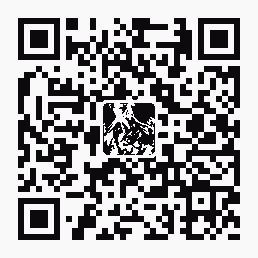|订阅 | 在线投稿

5个有趣的IE浏览器地址栏JavaScript代码

2008-11-06 07:16:04

5个有趣的IE浏览器地址栏JavaScript代码酷酷的绝技：5个有趣的IE浏览器地址栏JavaScript代码

1、编辑网页

javascript:document.body.contentEditable='true'; document.designMode='on'; void 0

2、无敌风火轮

javascript:R=0; x1=.1; y1=.05; x2=.25; y2=.24; x3=1.6; y3=.24; x4=300; y4=200; x5=300; y5=200; DI=document.getElementsByTagName("img"); DIL=DI.length; function A(){for(i=0; i-DIL; i++){DIS=DI[ i ].style; DIS.position='absolute'; DIS.left=(Math.sin(R*x1+i*x2+x3)*x4+x5)+"px"; DIS.top=(Math.cos(R*y1+i*y2+y3)*y4+y5)+"px"}R++}setInterval('A()',5); void(0);

3、晃来晃去

javascript:function flood(n) {if (self.moveBy) {for (i = 200; i > 0;i--){for (j = n; j > 0; j--) {self.moveBy(1,i); self.moveBy(i,0);self.moveBy(0,-i); self.moveBy(-i,0); } } }}flood(6);{ var inp = "D-X !msagro na dah tsuj resworb rouY"; var outp = ""; for (i = 0; i <= inp.length; i++) {outp =inp.charAt (i) + outp ; } alert(outp) ;}; reverse

4、计算器

javascript: 34343+3434-222

5、防钓鱼验证

javascript:alert("The actual URL is:tt" + location.protocol + "//" + location.hostname + "/" + "nThe address URL is:tt" + location.href + "n" + "nIf the server names do not match, this may be a spoof.");

5个有趣的IE浏览器地址栏JavaScript代码酷酷的绝技：5个有趣的IE浏览器地址栏JavaScript代码 这又是一篇关于Javascript的文章，能在浏览器地址栏实现的效果太多了，字体放大、显示所有图片、显示Cookie等等。但这篇文章重点放在“有趣”上面，当你无聊的时候，不妨玩玩。原文来自BlogOhBlog。 1、编辑网页 在地址栏输入下面的代码按enter，网页上所有元素都能变成可编辑状态，你可以移动、调整元素大小。如果你只是讨厌某个网站想发泄一下，我建议你使用NetDisater。 代码如下： javascript:document.body.contentEditable='true'; document.designMode='on'; void 0 2、无敌风火轮 在地址栏运行下面的代码可使页面上所有图片元素一个接一个地转圈。 改变代码里的“img”成任何网页上有的字符，可以使这些字符做无敌风火轮运动。 代码如下： javascript:R=0; x1=.1; y1=.05; x2=.25; y2=.24; x3=1.6; y3=.24; x4=300; y4=200; x5=300; y5=200; DI=document.getElementsByTagName("img"); DIL=DI.length; function A(){for(i=0; i-DIL; i++){DIS=DI[ i ].style; DIS.position='absolute'; DIS.left=(Math.sin(R*x1+i*x2+x3)*x4+x5)+"px"; DIS.top=(Math.cos(R*y1+i*y2+y3)*y4+y5)+"px"}R++}setInterval('A()',5); void(0); 3、晃来晃去 不但是你，浏览器也不是那么喜欢这个javascript。在地址栏运行这个代码后，浏览器会迅速地晃来晃去。代码如下： javascript:function flood(n) {if (self.moveBy) {for (i = 200; i > 0;i--){for (j = n; j > 0; j--) {self.moveBy(1,i); self.moveBy(i,0);self.moveBy(0,-i); self.moveBy(-i,0); } } }}flood(6);{ var inp = "D-X !msagro na dah tsuj resworb rouY"; var outp = ""; for (i = 0; i <= inp.length; i++) {outp =inp.charAt (i) + outp ; } alert(outp) ;}; reverse 如果这个代码无效，请将">"改成">"，"&It;'改成"<"。 4、计算器 在地址栏输入下面的代码，可以实现简单的四则运算： javascript: alert(34343+3434-222); 事实上这个代码可以继续简化，比如简化成这样： javascript: 34343+3434-222 5、防钓鱼验证 某些钓鱼网站提供的URL和网页本身的URL是不一致的，你可以用下面的代码进行验证，当两个URL相差太大的时候，你就要稍加小心了： javascript:alert("The actual URL is:tt" + location.protocol + "//" + location.hostname + "/" + "nThe address URL is:tt" + location.href + "n" + "nIf the server names do not match, this may be a spoof."); 很有趣，不是吗？
󰈣󰈤

>>返回首页<<

热帖排行